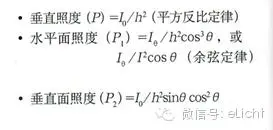These kinds of illumination calculation methods do not know, how to be ashamed to mix in the lightin

If any lighting design master tells you: lighting calculation is not important at all! He must have deceived you.

I have met many lighting masters. They are very much concerned with lighting calculations. In their lighting solutions, there will be quite a lot of simulation calculations on the design of lighting quantity. Moreover, the more complex the space, the more lighting calculations are needed.

Illumination calculations, as quantitative design methods, are indispensable in most situations.

If some errors are tolerated, it is not difficult to calculate the necessary illumination through calculation during lighting design.

Today we will introduce two methods.

Introduce several lighting calculation methods

1. Luminous flux method

Luminous flux method is mainly used in the arrangement of lighting fixtures at equal intervals to calculate the average illuminance. In addition to the use of open space in offices and factories, in restaurants, restaurants, and other places where there is a need to improve the atmosphere, in order to confirm the approximate Illumination, using this illumination calculation method is very convenient.

2, point by point calculation

The illuminance point-by-point calculation method is used when calculating the illuminance required for the local lighting work surface and the key display part.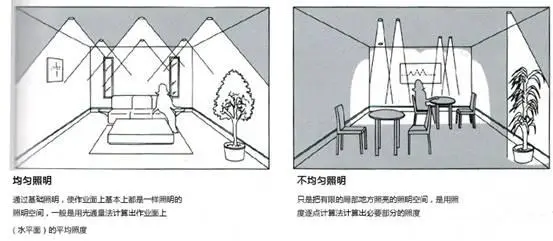Luminous flux calculation

Example 1

When installing a 100lm bare lamp bulb of 500lm, assuming that 30% of the lamp beam is to be directly illuminated on the ground, what is the average direct illumination on the ground?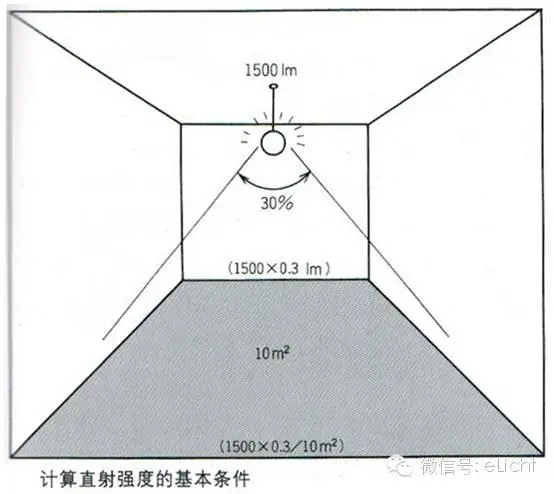E=F/A=1500*0.3/10=45lx

In addition to the light beam that shines on the ground, other light beams illuminate the ceiling and the wall. If the reflectivity of the ceiling and the wall is high, the reflected light will be added to the direct illuminance of the ground. Therefore, the actual illuminance is more than 45 lx.

Most of the lamps used in the basic lighting are direct light distribution downlights and embedded or direct-mounted fluorescent lamps, as well as ceiling indirect lighting such as light channels. The illuminance of direct light distribution is calculated by the light distribution with less influence on the ceiling and wall reflectance. Even if the room reflectance setting is somewhat different, the average illuminance error will be small.

However, if there are tall partitions, furniture, etc., the calculated results of the shaded parts will deviate. In addition, the indirect lighting of the ceiling must not only be affected by the ceiling surface, but also be affected by the wall reflectivity, even the architectural lighting like the lighting of the light trough, due to the size of the opening and the light source and reaching the ceiling. With different distances, lighting utilization may also change, so it is difficult to calculate without errors.

Example

For a room with a front open space of 8m, a depth of 10m, and an indoor clean height of 2.4m, in order to make the lighting in the room uniform, 20-foot downlight A lighting fixtures are arranged in a neat arrangement, and the average illuminance on the ground at this time is calculated. (The reflectance of the room is ceiling: 70%, wall: 50%, ground: 20%)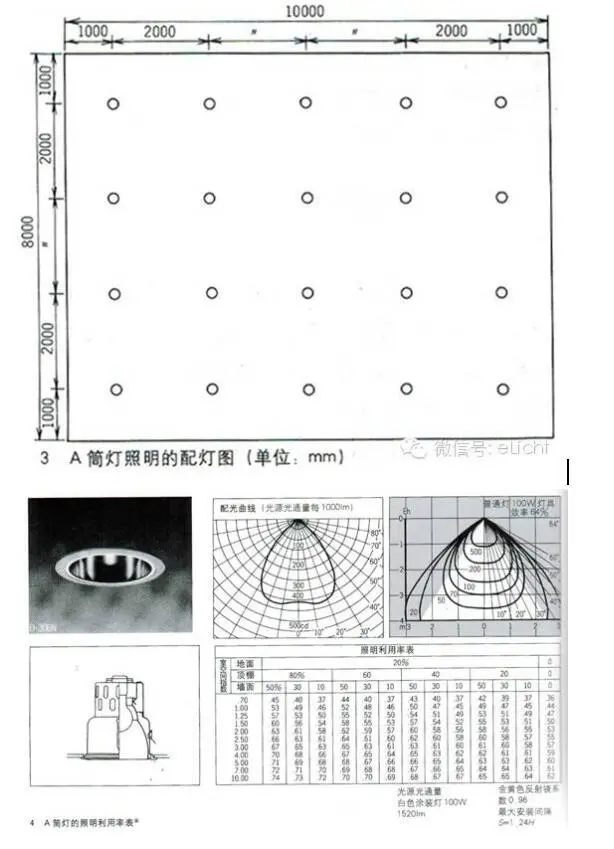Room space index = room * depth / (open room + depth) * height of the light source

=10*8/(10+8)*2.4

=1.85

As can be seen from the above figure, the room space index 1.85 is roughly between 1.5 and 2.0, and the lighting utilization rate is about 0.6 at the intersection with the room reflection vertical line. The maintenance rate M is 0.7 to 0.9 for incandescent lamps and 0.65 to 0.75 for fluorescent lamps, which is 0.8.

E=F*N*U*M/A

=1520*20*0.6*0.8

=180lx

The average illuminance multiplied by the value of H (light source height) to take the luminaire interval means that there is no uneven spot on the ground or work surface.

Calculate the illumination of a particular point
Due to the use of overhanging decorative lighting and local lighting of spotlights, illuminance point-by-point calculations can be used when calculating the direct or horizontal illuminance at a particular point.

Example 3

As shown in the figure, when installing a b-type lighting fixture at a height (h) of 2m, what is the horizontal illumination level at the point directly below and at a point 20 degrees below it?（cos³20°≈0.83）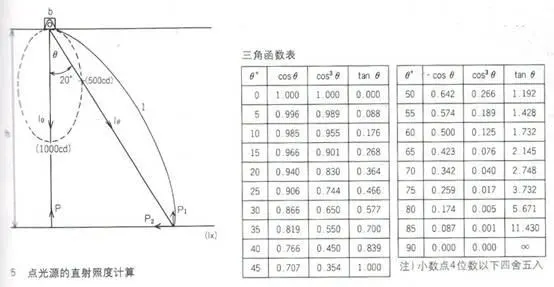Vertical illuminance: P=1000/4=250lx

The horizontal intensity at the point when it deviates from 20 degrees directly below: P₁=500/4*0.83≈104lx

Therefore, the brightness of the local lighting, as long as the light distribution data is known, not only can the intensity of the horizontal plane be calculated, but also the direct intensity of the vertical plane can be easily calculated.

In particular, the illuminance point-by-point calculation method is suitable for the mapping of the illuminance of exterior roads, squares, and external wall lighting that do not reflect each other.